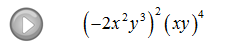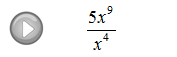## Pages

### Rules of Exponents

Before learning how to add, subtract, multiply and divide polynomials we need to understand the rules of exponents.

There will be many new rules to remember and you might feel overwhelmed. However, with much practice you will quickly learn to use them effortlessly.  (In this section we will assume all variables are real and exponents are integers.)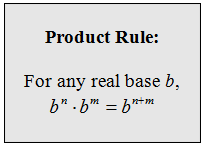When multiplying two real numbers with the same base you add exponents.Multiply.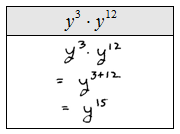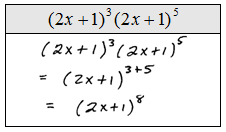Add exponents only when the bases are exactly the same.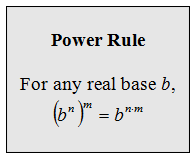When raising an exponent to a power multiply the exponents.Simplify.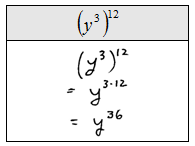Never multiply a base with an exponent.When we raise a product to a power we must raise each factor to that power.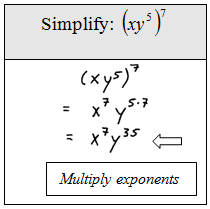Simplify.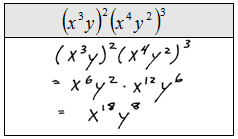Next we consider the power of a quotient.When raising a quotient to a power, raise numerator and denominator to that power. Assume the variables in the denominator are nonzero. If we have zero in the denominator then the expression is undefined.Simplify.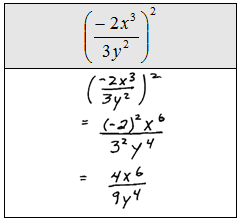If you raise expressions to an even power then the quantity will be positive. If you raise negative expressions to an odd power then the quantity will be negative.When dividing two expressions with the same base we subtract exponents. This rule is basically cancellation in disguise.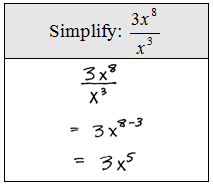Simplify (Assume all variables are nonzero.)Next we cover the zero exponent rule.When an expression is raised to the zero power it will be equal to 1, unless the base is zero. Zero to the zero power is undefined.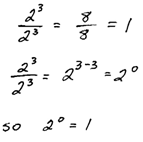Simplify (Assume all variables are nonzero.)